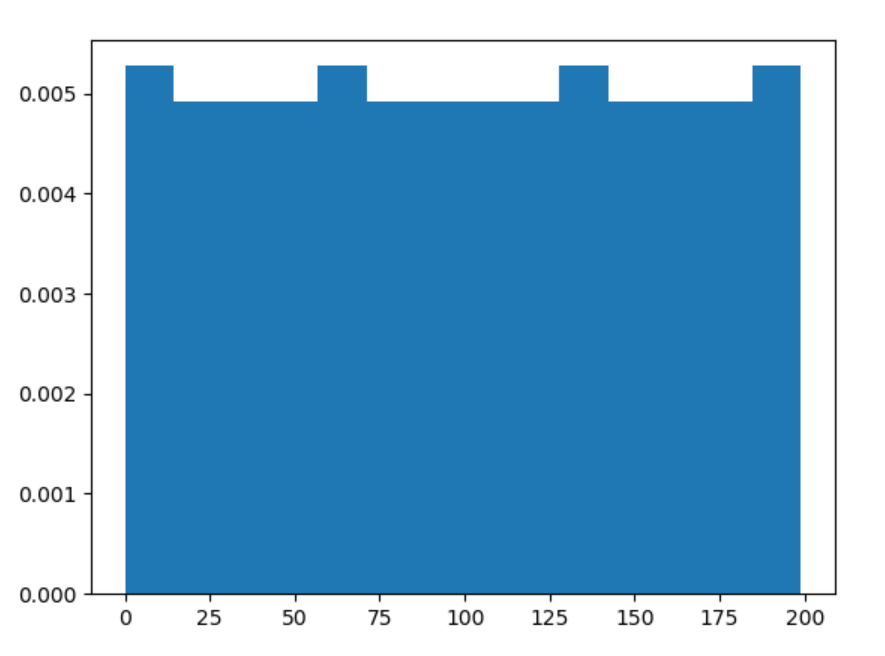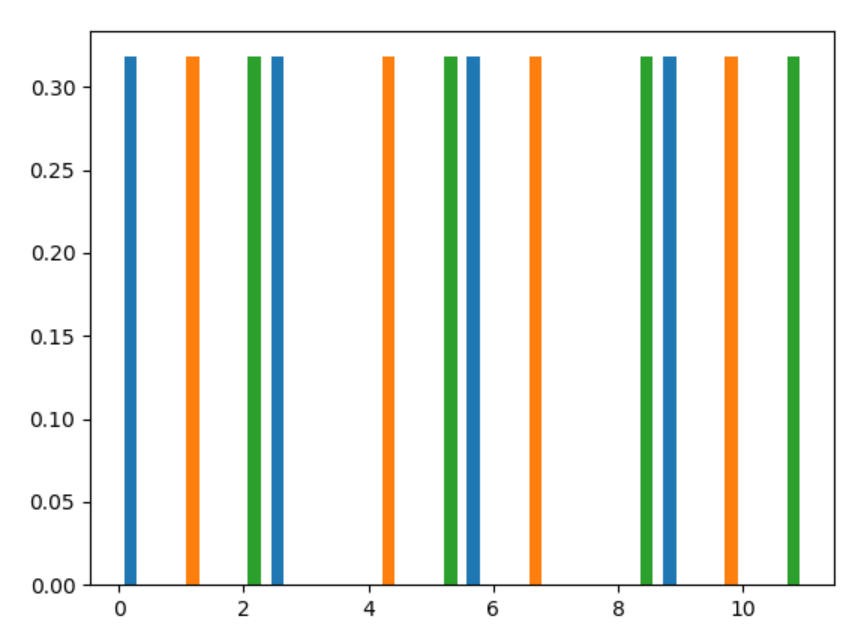# numpy.random.permutation() in Python

• Last Updated : 15 Jul, 2020

With the help of numpy.random.permutation() method, we can get the random samples of sequence of permutation and return sequence by using this method.

Syntax : numpy.random.permutation(x)

Return : Return the random sequence of permuted values.

Example #1 :

In this example we can see that by using numpy.random.permutation() method, we are able to get the sequence of permutation and it will return the sequence by using this method.

## Python3

 `# import numpy``import` `numpy as np``import` `matplotlib.pyplot as plt`` ` `# Using permutation() method``gfg ``=` `np.random.permutation(``200``)`` ` `count, bins, ignored ``=` `plt.hist(gfg, ``14``, density ``=` `True``)``plt.show()`

Output :Example #2 :

## Python3

 `# import numpy``import` `numpy as np``import` `matplotlib.pyplot as plt`` ` `arr ``=` `np.arange(``12``).reshape((``4``, ``3``))``# Using permutation() method``gfg ``=` `np.random.permutation(arr)`` ` `count, bins, ignored ``=` `plt.hist(gfg, ``14``, density ``=` `True``)``plt.show()`

Output :My Personal Notes arrow_drop_up